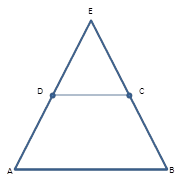Chapter 4.4, Problem 18E### Elementary Geometry for College St...

6th Edition
Daniel C. Alexander + 1 other
ISBN: 9781285195698

#### Solutions

Chapter
Section### Elementary Geometry for College St...

6th Edition
Daniel C. Alexander + 1 other
ISBN: 9781285195698
Textbook Problem
1 views

# Given: Isosceles Δ A B E with A E ¯ ≅ B E ¯ ; also, D and C are midpoints of A E ¯ and B E ¯ , respectively.Prove: ABCD is an isosceles trapezoid.Exercises 17, 18

To determine

To Prove:

The trapezoid is isosceles.

Explanation

Consider the following diagram.

Given: Isosceles ΔABE with AE¯BE¯, also D and C are midpoints of AE¯ and BE¯, respectively.

Prove: ABCD is an isosceles trapezoid

### Still sussing out bartleby?

Check out a sample textbook solution.

See a sample solution

#### The Solution to Your Study Problems

Bartleby provides explanations to thousands of textbook problems written by our experts, many with advanced degrees!

Get Started

#### Use the guidelines of Section 4.5 to sketch the curve. y=x1x2

Single Variable Calculus: Early Transcendentals, Volume I

#### Let h be the function defined by h(x) = x3 x2 + x + 1. Find h(5), h(0), h(a), and h(a).

Applied Calculus for the Managerial, Life, and Social Sciences: A Brief Approach

#### is the binomial series for:

Study Guide for Stewart's Single Variable Calculus: Early Transcendentals, 8th